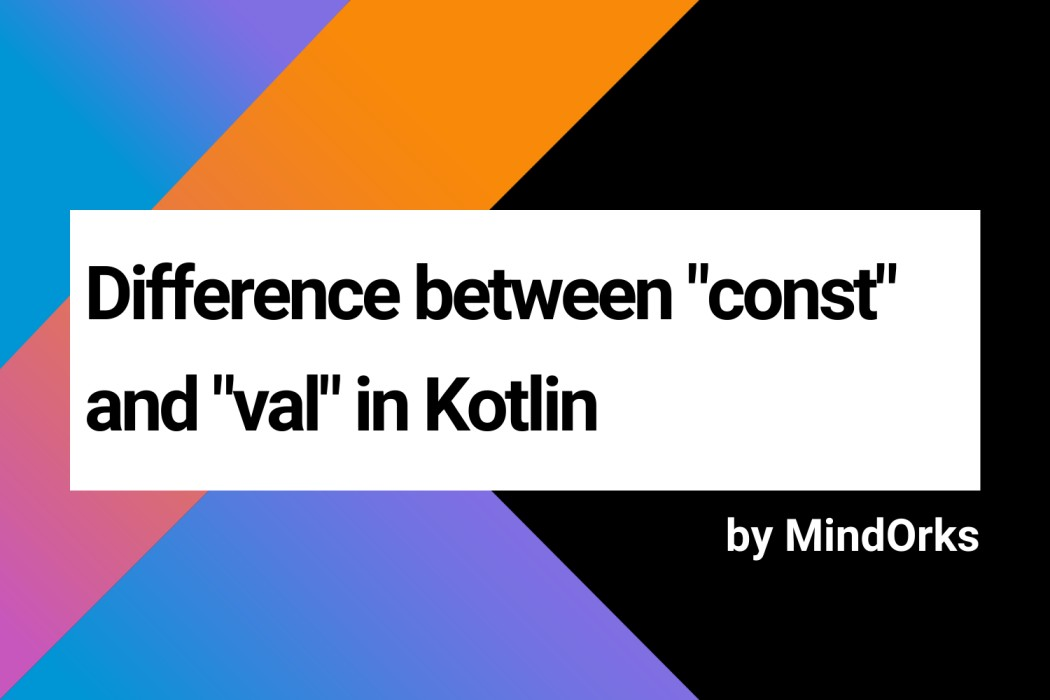# What is the difference between "const" and "val"?In Kotlin, as like other programming languages, the properties can either be mutable(it can be changed) or immutable(it can't be changed). We use the keywords ``` const ``` and ``` val ``` to declare an immutable property . Developers often get confused between these terms i.e. when to use which one.

So, welcome to MindOrks, in this tutorial, we will see the difference between ``` const ``` and ``` val ``` in Kotlin.

#### Use of "const" in Kotlin

The ``` const ``` keyword is used to declare those properties which are immutable in nature i.e. these properties are read-only properties.

But, the value of these properties must be known at the compile-time only and this is the reason ``` const ``` is also known as Compile-time constant. So, no runtime assignment of values is allowed in ``` const ``` variables.

A property must satisfy the following to be a ``` const ``` property:

• must be at top-level or member of object or member of a companion object
• must be initialised with a ``` String ``` type or primitive type
• no custom getter

So, you can't assign a ``` const ``` variable to a function or to some class because in this case the variable will be initialised at the runtime and not at compile-time.

#### Use of "val" in Kotlin

The ``` val ``` keyword is also used for read-only properties. But the main difference between the ``` const ``` and ``` val ``` is that the ``` val ``` properties can be initialised at the runtime also.

So, you can assign a ``` val ``` variable to a function or to some class.

#### Example

``````const val companyName = "MindOrks" // this will work
val comapanyname = "MindOrks" // this will work

const val companyName = getCompanyName() // will not work
val companyName = getCompanyName() // this will work``````

In the above example, we are using ``` companyName ``` as the immutable variable. When using ``` const ``` , if you directly assign the value then it is fine but if you try to assign the value from some function ``` getCompanyName ``` , then you will get an error because here the value will be assigned at runtime and not at compile-time. But in the case of ``` val ``` , both the situations are ok.

#### Why use "const" when we can use "val"?

In the previous example, we saw that the value of the ``` val ``` variable is initialized at runtime and ``` const ``` at compile time. So, why to use ``` const ``` if we can use ``` val ``` ?

Let's find by taking another real-life Android example to understand the use-case of ``` const ``` and ``` val ``` :

``````YourClassName {
companion object {
const val FILE_EXTENSION = ".png"
val FILENAME: String
get() = "Img_" + System.currentTimeMillis() + FILE_EXTENSION
}
}``````

In the above example, we are declaring the ``` const ``` variable named FILE_EXTENSION in the companion object and the ``` FILENAME ``` variable as ``` val ``` and initialise it with custom getter.

As the extension of the file will always be same, so it is declared as a ``` const ``` variable. But, the name of the file will be changed based on the logic that we use for the file name. Here, in our example, we are naming the file based on the current time. You can't give some value to it initially because the value is fetched at the runtime. So, we are using ``` val ``` here.

What happens after the code compilation is that wherever the ``` const ``` variables are used in the code, those variables are replaced by the value of that ``` const ``` variable but in case of ``` val ``` , the variables are kept as it is because we don't know the value of ``` val ``` at compile-time. So, if you decompile the above code, then you will see( learn how to covert Kotlin code into Java ):

``````public final String getFILENAME() {
return "Img_" + System.currentTimeMillis() + ".png";
}``````

Here, you can find that the variable ``` FILE_EXTENSION ``` has been replaced by its value i.e. ".png" i.e. the value has been inlined and hence there is no overhead to access that variable at the runtime. This is the advantage of using ``` const ``` over ``` val ``` .

This is all about the difference between ``` const ``` and ``` val ``` keyword in Kotlin.